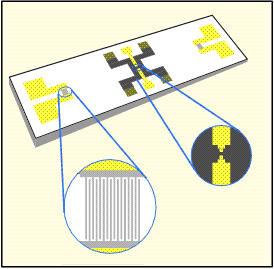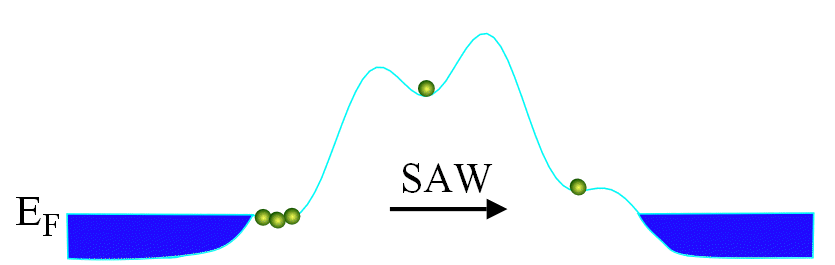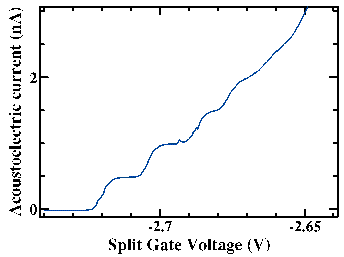Semiconductor Physics Group

## SAW-driven quantised charge transport

This project has been attempting to develop a usable standard of electrical current. The S.I. definition of the ampere is still "That current which, when flowing through two parallel infinite wires held one metre apart will induce between the wires a force of 2*10-7N/m of length". A much better definition would be one based on the flow of a known number of electrons through a system.

Several results have been published on devices which "pump" one electron at a time through the circuit, giving a current equal to ef, where e is the charge on an electron and f is the pumping frequency. All of these devices have relied on the same principle, that of Coulomb Blockade by an isolated region of electrons (known as a quantum dot). These are generally defined electrostatically using surface gates, with electrons able to move in and out of the quantum dot across tunnel barriers. Lowering the entrance barrier allows a single electron to tunnel onto the dot. The entrance barrier is then raised, and the exit lowered so an electron may move off the dot on the other side of the device. The reliance on quantum-mechanical tunnelling limits the frequency of operation of these "electron turnstiles" to about 10MHz. This corresponds to a current of a few picoamps, which is too small to be measured to the required level of accuracy (parts per billion) at the moment. However researchers have demonstrated that the devices are stable to this level, and attempts are being made to improve the accuracy of the measurements.

Our approach is rather different. Instead of using electrostatic gates to confine the electrons in a dot, we use a combination of metal side gates and the piezoelectric field associated with a moving surface acoustic wave (SAW).Figure 3: SAW quantised charge pump device.

The diagram shows one of the devices used in our experiments. The contacts for the inter-digitated transducers (IDTs) used to generate SAWs are at either end of the chip, with the mesa and 2DEG in between. The inset on the left shows one of the transducers. When r.f. power is applied at the correct frequency, given by f=vSAW/lIDT, then strong SAWs are produced. The best IDTs we can produce at present have a repeat unit of 1µm, with an excitation frequency of 2.8GHz. The SAWs move over the surface in a narrow beam towards the second IDT. As they move, the mechanical movement generates an electrostatic field. The 2DEG region is shown in black. The electrons interact with the electrostatic field of the SAW, and are dragged along, giving a current. This is known as the acoustoelectric current.

The second inset shows the split gate in the centre of the device. Applying a negative voltage to the two arms of the gate repels electrons from the 2DEG beneath. Current can now only flow in the small channel below the gap. If the negative voltage is large enough, and the power in the SAW is high, then a quantum dot is formed, with transverse confinement due to the split gate, and longitudinal confinement due to the SAW.

The crucial difference between our devices and electron turnstiles is that the dots move with the SAW. As the SAW moves across the split gate it traps a patch of electrons in the potential minimum. This patch then moves across the split gate, shrinking to form a quantum dot as the lateral confinement increases. Shrinking the dot forces some of the electrons out due to Coulomb Blockade. When the dot is in the centre of the split gate, there is only one electron left (see the figure below). This moves to the other side of the constriction with the SAW. This process is repeated for each SAW cycle, giving a current equal to ef.Figure 4. Animation of electrons being dragged over a potential hill.

Below is a plot of the acoustoelectric current generated in one of these devices as the voltage on the split gate arms is reduced. A current staircase is formed as first one electron is transferred per cycle, then two etc.Figure 5: SAW acoustoelectric current.

The present definitions of the volt and ohm already used quantum-mechanical effects. The volt is defined in terms of the a.c. Josephson effect, and the ohm in terms of the quantum Hall effect. If we can use the devices above to define the ampere, then it will finally be possible to "close the metrological triangle" of electrical units.